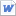검색어 입력폼

# concepts in Thermal physics-introduction,heat

저작시기 2014.09 |등록일 2014.11.24MS 워드 (docx) | 3페이지 | 가격 600원

1. Introduction
2. Heat

## 본문내용

1. Introduction
Molar mass: mass of one mole of the substance

“In the thermodynamic limit”
Suppose that container of gas has volume V, temperature T, pressure P, and kinetic energy of all the gas molecules adds up to U. Let’s call the volume of this half of gas V*,
V*=V/2, U*=U/2 but p*=p, T*=T
Extensive variables: scale with the system size, like V and U
Intensive variables: independent of system size, like p and T
-classical thermodynamics deals with macroscopic projects, without worrying about the underlying microscopic physics.

없음